Search

Exponential Functions: Introduction (page 1 of 5)

Sections: Introduction, Evaluation, Graphing, Compound interest, The natural exponentialExponential functions look somewhat similar to functions you have seen before, in that they involve exponents, but there is a big difference, in that the variable is now the power, rather than the base. Previously, you have dealt with such functions as f(x) = x2, where the variable x was the base and the number 2 was the power. In the case of exponentials, however, you will be dealing with functions such as g(x) = 2x, where the base is the fixed number, and the power is the variable.

Let's look more closely at the function g(x) = 2x. To evaluate this function, we operate as usual, picking values of x, plugging them in, and simplifying for the answers. But to evaluate 2x, we need to remember how exponents work. In particular, we need to remember that negative exponents mean "put the base on the other side of the fraction line".

 So, while positive x-values give us values like these: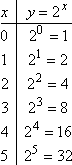...negative x-values give us values like these:     Copyright © Elizabeth Stapel 2002-2011 All Rights Reserved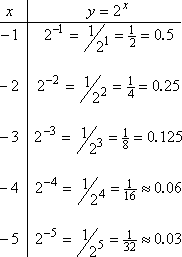Putting together the "reasonable" (nicely graphable) points, this is our T-chart:...and this is our graph: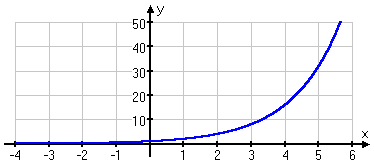You should expect exponentials to look like this. That is, they start small very small, so small that they're practically indistinguishable from "y = 0", which is the x-axis and then, once they start growing, they grow faster and faster, so fast that they shoot right up through the top of your graph.

You should also expect that your T-chart will not have many useful plot points. For instance, for x = 4 and x = 5, the y-values were too big, and for just about all the negative x-values, the y-values were too small to see, so you would just draw the line right along the top of the x-axis.

Note also that my axis scales do not match. The scale on the x-axis is much wider than the scale on the y-axis; the scale on the y-axis is compressed, compared with that of the x-axis. You will probably find this technique useful when graphing exponentials, because of the way that they grow so quickly. You will find a few T-chart points, and then, with your knowledge of the general appearance of exponentials, you'll do your graph, with the left-hand portion of the graph usually running right along the x-axis.

You may have heard of the term "exponential growth". This "starting slow, but then growing faster and faster all the time" growth is what they are referring to. Specifically, our function g(x) above doubled each time we incremented x. That is, when x was increased by 1 over what it had been,  y increased to twice what it had been. This is the definition of exponential growth: that there is a consistent fixed period over which the function will double (or triple, or quadruple, etc; the point is that the change is always a fixed proportion). So if you hear somebody claiming that the world population is doubling every thirty years, you know he is claiming exponential growth.

Exponential growth is "bigger" and "faster" than polynomial growth. This means that, no matter what the degree is on a given polynomial, a given exponential function will eventually be bigger than the polynomial. Even though the exponential function may start out really, really small, it will eventually overtake the growth of the polynomial, since it doubles all the time.

 For instance, x10 seems much "bigger" than 10x, and initially it is: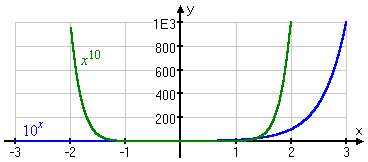But eventually 10x (in blue below) catches up and overtakes x10 (at the red circle below, where x is ten and y is ten billion), and it's "bigger" than x10 forever after: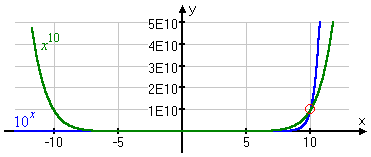Exponential functions always have some positive number other than 1 as the base. If you think about it, having a negative number (such as 2) as the base wouldn't be very useful, since the even powers would give you positive answers (such as "(2)2 = 4") and the odd powers would give you negative answers (such as "(2)3 = 8"), and what would you even do with the powers that aren't whole numbers? Also, having 0 or 1 as the base would be kind of dumb, since 0 and 1 to any power are just 0 and 1, respectively; what would be the point? This is why exponentials always have something positive and other than 1 as the base.

Top  |  1 | 2 | 3 | 4 | 5  |  Return to Index  Next >>

 Cite this article as: Stapel, Elizabeth. "Exponential Functions: Introduction." Purplemath. Available from     https://www.purplemath.com/modules/expofcns.htm. Accessed [Date] [Month] 2016

Study Skills Survey

Tutoring from Purplemath
Find a local math tutor